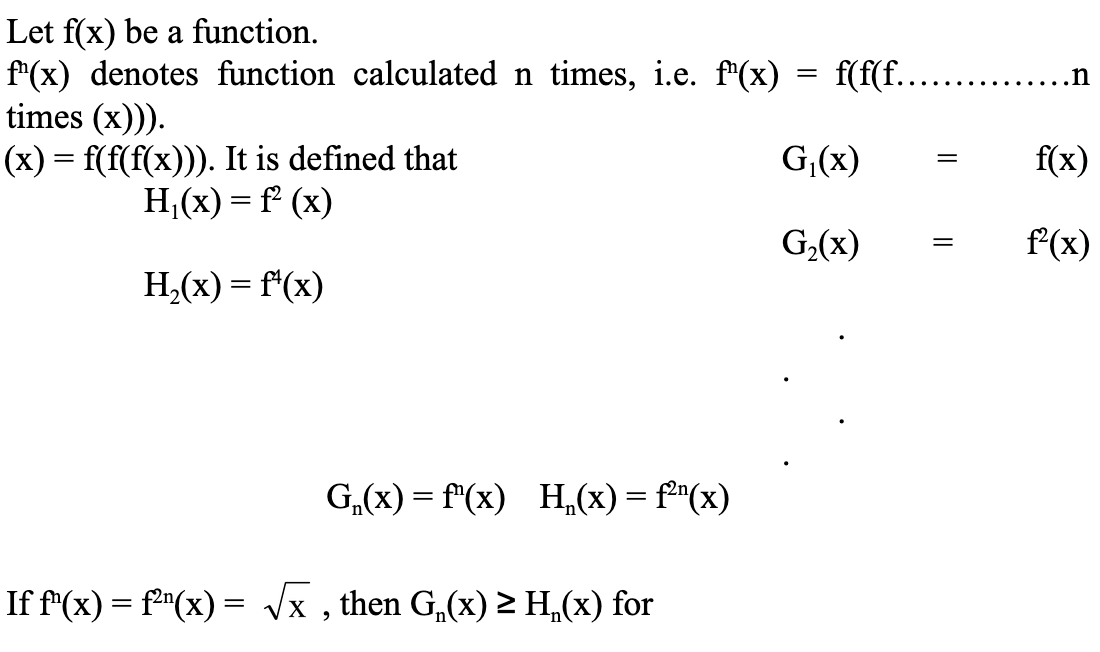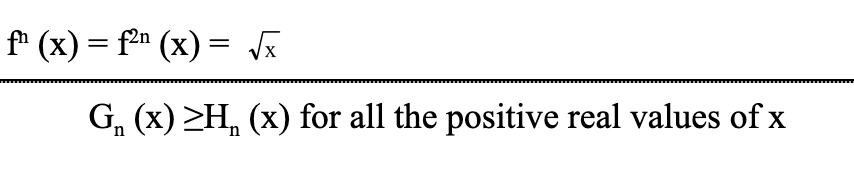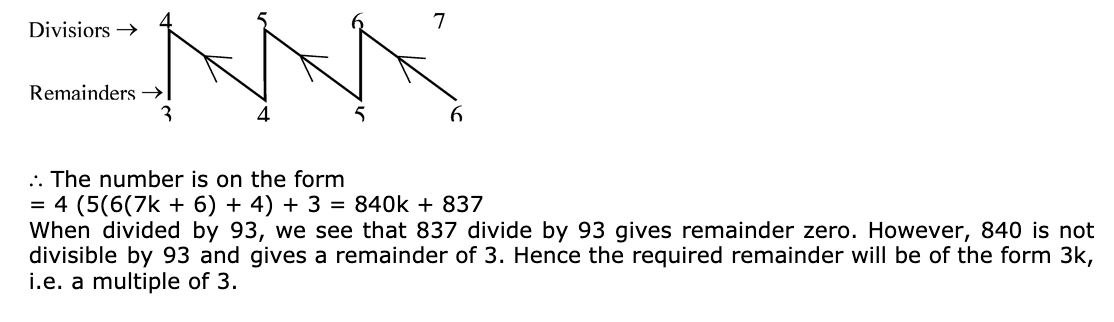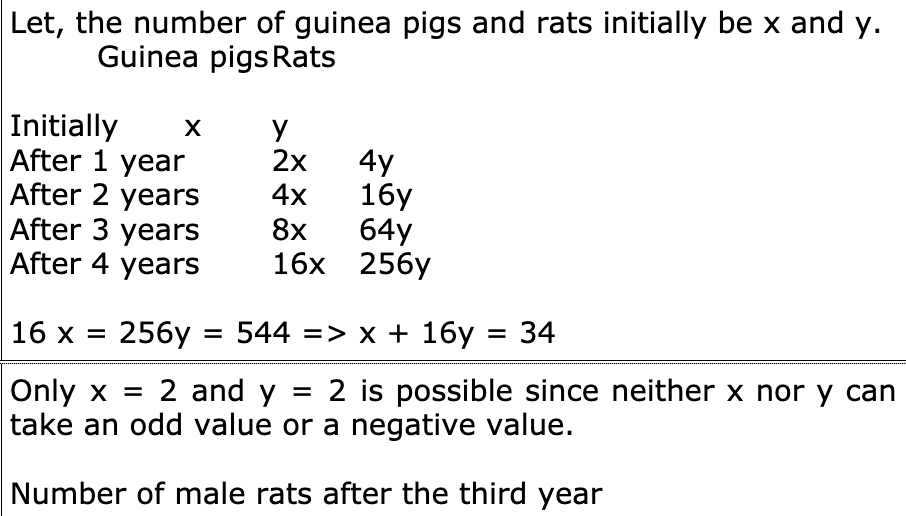Jobs By Batch
Jobs By Location
Jobs By Degree
Jobs By Branch
IT Jobs
Internships
Govt. Jobs• ##### Original Questions of IT Companies| All TopicsQ #6
:

#### Quants Question

Read the following data to answer the questions given below Let f(x) be a function. fn(x) denotes function calculated n times, i.e. fn(x) = f(f(f�����n times (x))). So f3(x) = f(f(f(x))). It is defined that G1(x) = f(x) H1(x) = f2 (x) G2(x) = f2(x) H2(x) = f4(x) . . . . Gn(x) = fn(x) Hn(x) = f2n(x) If fn(x) = f2n(x) = , then Gn(x) >= Hn(x) for+Q #7
:

#### Quants Question

Directions : Select the correct alternative from the given choices.

A number when successively divided by 4, 5, 6 and 7 leaves remainders of 1, 4, 5 and 6 respectively. Which of the following can be the remainder when the above number is divided by 93?
+Q #8
:

#### Quants Question

Directions : These questions are based on the data given below.

This game is played by two people. Put 18 dots on a black board or on paper. Each player is to erase 1, 2 or 3 dots in his playing turn. Turns alternate between the two players, i.e. player 1 takes turn 1, then player 2 takes turn 2, then player 1 takes turn 3, and so on. You need not chose a number and stay with it. For instance, you might erase 1 dot on your firs turn, 2 dots on your second, 1 dot on your third and 3 dots on your fourth turn. The player who erases the last dot loses. You make the first move, and both players play intelligently, and you win. What is the number of dots that were erased in turns 1, 2 and 3 together?
+

Always 1 less in the last move. And for each successive move, less is (maximum that can be drawn + 1) Hence 19, 15, 11 and 7. (Note that it is because every move made by 1 can be squared off to 4. i.e. 1 + maximum draw).

Q #9
:

#### Quants Question

Directions : These questions are based on the data given below.

N! (N >= 2004) is divided by 10th to leave a remainder of r(r >= 0). If m is the maximum possible are r is the minimum possible, then the last digit of the quotient is
+

Here, N = 2004! is divided by the largest power of 10 that perfectly divides it. Hence all the zeroes from the end are removed and the last digit then is what is required. Since the highest power of 2 that divides 2004! is definitely greater than the highest power of 5 that divides 2004! ( 10 = 2 x 5), even after removing all zeroes, the quotient will still be even. Therefore The last digit of the quotient is always even irrespective of the parity (even or odd) of m.

Q #10
:

#### Quants Question

Directions : These questions are based on the data given below.

Dr. Surya Rao is working on an experiment since the last four years. He is carrying out the experiment on guinea pigs and rats. The number of guinea pigs doubled every year while the rats quadrupled every year. In the last four years that Dr. Rao has been doing the experiments, he found that the mortality rat of both guinea pigs and rats was nil and their total population grew to 544. From the beginning the number of males and females had always been equal. How many male rats were there after the third year?
+